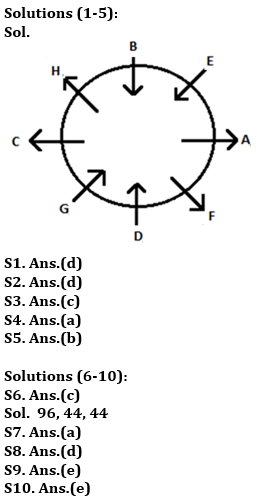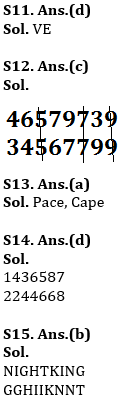Latest Banking jobs   »   Revision Test Reasoning Ability Quiz For...

# Revision Test Reasoning Ability Quiz For IBPS RRB PO, Clerk Prelims 2021- 6th June

Directions (1-5): Study the following information carefully and answer the given questions.
Eight students have seated around a circular table in which some are facing towards the centre and some are facing outside the centre. E sits third to the right of D. Only two persons sit between G and B, both are facing same direction. A sits third to the right of the one who sits second to the left of G. F is neither immediate neighbour of G nor B. C sits third to the right of F who sits opposite to H. G is facing same direction as D but opposite to C and A. E sits second to the right of H who is facing same direction as F. B and D faces each other. E faces towards the center.

Q1. Who among the following sits third to the right of A?
(a) E
(b) F
(c) D
(d) G
(e) None of these

Q2. Who among the following sits opposite to C?
(a) B
(b) E
(c) H
(d) A
(e) None of these

Q3. How many persons sits between D and H, when counting left of D?
(a) Four
(b) Three
(c) Two
(d) One
(e) None

Q4. What is the position of B with respect to F?
(a) Third to the left
(b) Immediate right
(c) Second to the right
(d) Immediate Left
(e) None of these

Q5. How many persons facing inside?
(a) One
(b) Four
(c) Three
(d) Two
(e) None of these

Directions (6-10): Study the following number sequence and answer the questions following it.

8 1 3 2 5 4 7 6 8 9 6 1 3 5 2 8 4 4 4 5 2 7 8 2 9 7 2 1 5 6

Q6. How many even numbers are there in the numeric series which are immediately preceded by a number, which is a whole square?
(a) One
(b) Two
(c) Three
(d) More than three
(e) None of these

Q7. If all the odd numbers are dropped from the series, which number will be fifth to the right of seventh number from the left end?
(a) 2
(b) 8
(c) 6
(d) 4
(e) None of these

Q8. If all 1 is replaced by 2 and all 4 is replaced by 5 in the given number series, then which number will be fourth to the right of seventeenth number from the right end?
(a) 1
(b) 2
(c) 3
(d) 5
(e) 6

Q9. Which of the following number will be 8th to the right of 17th number from the right end?
(a) 5
(b) 6
(c) 8
(d) 9
(e) None of these

Q10. How many odd number/s which is/are immediately preceded by a ‘perfect square’ and immediately followed by a perfect cube are there in the above sequence?
(a) One
(b) Two
(c) Three
(d) More than three
(e) None

Q11. What should come in place of question mark (?) in the following series based on the above arrangement?
ZA  YB  XC  WD  ?
(a) EU
(b) LO
(c) LP
(d) VE
(e) None of these

Q12. How many number will be remain at the same position in the given number “46579739” when they are arranged in the ascending order from left to right?
(a) One
(b) Two
(c) Three
(d) More than three
(e) None of these.

Q13. If it is possible to make only one meaningful word with the 1st ,2nd ,4th and 7th letters of the word ‘CAREERPOWER’ which would be the third letter of the word from the right? If more than one such word can be formed give ‘Y’ as the answer. If no such word can be formed, give ‘Z’ as your answer.
(a) Y
(b) P
(c) C
(d) Z
(e) A

Q14. If 1 is added to each odd digit and 2 is subtracted from each even digit in the number 1436587, then how many digits will appear twice in the new number thus formed?
(a) Only 8
(b) Only 8 and 6
(c) 8, 6 and 4
(d) 2, 4 and 6
(e) None of these

Q15. How many letters will be remaining at the same position in the word ‘NIGHTKING’ when letters arranged according to alphabetical order from left to right?
(a) One
(b) Two
(c) Three
(d) More than Three
(e) None

Practice More Questions of Reasoning for Competitive Exams:

###### Study Plan for IBPS RRB PO/Clerk Prelims 2021

Solutions